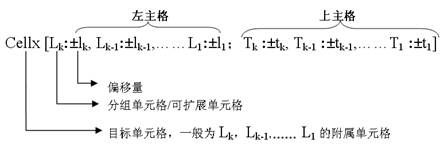# 扩展区域与静态区域混合（1） E3单元格输入表达式：=sum(D3{D3>10000})

（2） E5单元格输入表达式：=sum(D5{D5>10000})

（3） D4单元格输入表达式：=sum(D3{D3>10000})

（4） D6单元格输入表达式：=sum(D5{})

（5） G3单元格输入表达式：=sum(F3{})

（6） G5单元格输入表达式：=sum(F5{})

（7） F4单元格输入表达式：=sum(F3{F3>10000})

（8） F6单元格输入表达式：=sum(F5{})

E3格中的表达式为：=sum(D3{D3>10000})。其含义是：订购日期为C3的且销售额大于1000的之和；

D3格中的表达式为：=sum(F3{})。其含义是：订购日期为C3的销售额之和。